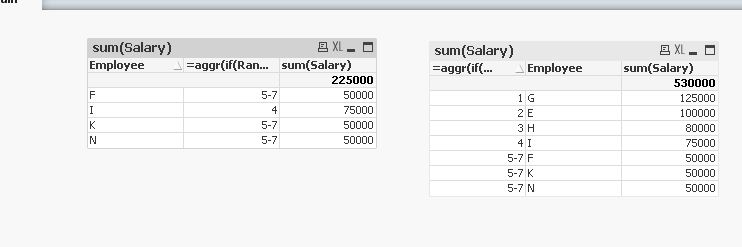# QlikView App Dev

Discussion Board for collaboration related to QlikView App Development.

Announcements
Customer & Partners, DEC. 9, 11 AM ET: Qlik Product & Strategy Roadmap Session: Data Analytics REGISTER NOW
cancel
Showing results for
Did you mean:Not applicable

## Ranking - Top 20 Next 20

Hi

I have written a Sales Rep report that emails out a Top 20 Sales People graph in N Printing - Known as Division 1 Sales.

I am now being asked to create a Division 2 Sales table, how in Qlik is it possible to create a table looking at the Ranked Sales 21 - 40

I assume that Ranking has something to do with it but not sure how to use this function.

Any help would be gratefully received.

Thanks

Dave

1 Solution

Accepted SolutionsPartner

Regarding the performance I'd recommend using set analysis, you could try it with this expression:

SUM({<Employee = {"=Rank(SUM(Salary))>20"} * {"=Rank(SUM(Salary))<=40"}>}Salary)

Regards,

Patrick

Edit 19.09.17: as kush141087‌ mentioned, the intersection is not as performant as the "and" operator:

Therefore

SUM({<Employee = {"=Rank(SUM(Salary))>20 and Rank(SUM(Salary))<=40"}>}Salary)

should perform better

24 RepliesMVP

you can try expression something like

=if(rank(sum(Sales),0,1)>=21 and rank(sum(Sales),0,1)<= 40,sum(Sales))Master II

Hi,

Try like this,

=aggr(if(Rank(sum(Salary))>=21 and Rank(sum(Salary))<=40,Rank(sum(Salary))),Employee)MVP

If you have used a rank() in expression for creating the top 20 chart, the same expression could be modified for the 21-40 ranked sales. Say you have used expression something like:

=Sum({<SalesMan={"=Rank(Sum(Sales))<=20"}>}Sales)

for 21-40, modifiy it to something like:

=Sum({<SalesMan={"=Rank(Sum(Sales))>20 and Rank(Sum(Sales))<=40"}>}Sales)Partner

Regarding the performance I'd recommend using set analysis, you could try it with this expression:

SUM({<Employee = {"=Rank(SUM(Salary))>20"} * {"=Rank(SUM(Salary))<=40"}>}Salary)

Regards,

Patrick

Edit 19.09.17: as kush141087‌ mentioned, the intersection is not as performant as the "and" operator:

Therefore

SUM({<Employee = {"=Rank(SUM(Salary))>20 and Rank(SUM(Salary))<=40"}>}Salary)

should perform betterMVP

As i know there is no function Rank() in script, But if really want to achieve then i would prefer like below

Fact:

Name, Sales

Rajendra, 10

Anil, 20

Weel, 30

];

Fact_Copy:

sum(Sales) as Total_Sales

Resident Fact

GROUP BY Name;

Fact_S_Copy:

RowNo() as Rank,

Total_Sales

RESIDENT Fact_Copy

ORDER BY Total_Sales asc;

DROP TABLE Fact_Copy;

Table:

Total_Sales,

if( Peek('Total_Sales') = Total_Sales, Peek('Dense_Rank'), Rank) as Dense_Rank

RESIDENT Fact_S_Copy

ORDER BY Total_Sales asc;

DROP TABLE Fact_S_Copy;

DROP Field Sales;

Before develop something, think If placed (The Right information | To the right people | At the Right time | In the Right place | With the Right context)MVP

or better use set analysis

=sum({<SalesRep ={"=rank(sum(Sales),0,1)>=21 and rank(sum(Sales),0,1)<= 40"}>} Sales)Partner

Isn't the problem in this case that you precalculate a rank which doesn't react to selections.
Therefore selecting a specific Product group wouldn't actually give the correct rank for this group but for the whole precalculation?MVP

Hello Dave,

Have you checked and sure the expression provided by Patrick really works? You marked it correct, however, the expression looks not correct to me.MVP

I thought, I've replied for this..

 I am now being asked to create a Division 2 Sales table, how in Qlik is it possible to create a table looking at the Ranked Sales 21 - 40
Before develop something, think If placed (The Right information | To the right people | At the Right time | In the Right place | With the Right context)Community Browser